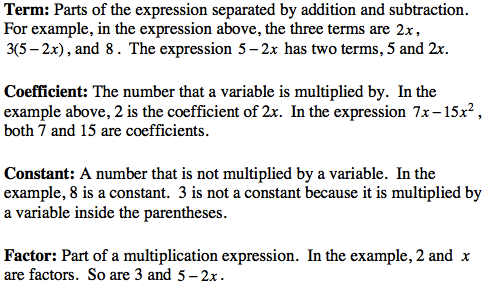Home > CC2MN > Chapter cc17 > Lesson cc17.3.3 > Problem7-107

7-107.

Identify the terms, coefficients, constant terms, and factors in each expression below. Homework Help ✎

1. $3x^2 + (−4x) + 1$

Refer to the vocabulary from the Math Notes box in Lesson 7.3.3 below.

1. $3(2x − 1) + 2$

Terms: $3(2x − 1)$ and $2$
Coefficients: $3$ and $2$
Constant term: $2$
Factors: $3$ and $(2x −1)$